If Sin A = 3/4, Calculate cos A and tan A

• Last Updated : 18 Oct, 2021

Trigonometry is one of the branches of Mathematics. It is the study of the property of triangles like it is the relation between lengths of its sides and angles between them. The ratios involved in the trigonometric problems are called trigonometric ratios. Trigonometry helps in understanding any topic that involves distances, angles, waves problems, etc. The trigonometric functions (sin, cos, and tan) are key to study science, technology, and engineering.

The above-given problem is simple and is solved using trigonometric ratios and the Pythagoras theorem.

Trigonometric ratios

Trigonometric ratios are the very beginning thing to learn in trigonometry. These ratios from the basic foundation required to understand this branch of mathematics. Here are all the Trigonometric ratios that we are talking about,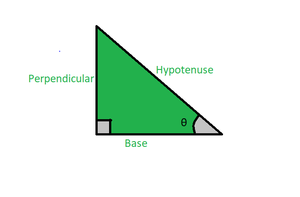Right-angled Triangle

sin(θ) = Perpendicular/Hypotenuse

cos(θ) = Base/Hypotenuse

tan(θ) = Perpendicular/Base

cot (θ) = 1/tan (θ) = Base/Perpendicular

sec (θ) = 1/cos (θ) = Hypotenuse/Base

cosec (θ) = 1/sin (θ) = Hypotenuse/Perpendicular

Pythagoras Theorem

The Pythagoras Theorem states that if a triangle is a right angled triangle then the sum of squares of perpendicular and base of the triangle is equal to the square of a hypotenuse of a triangle i.e.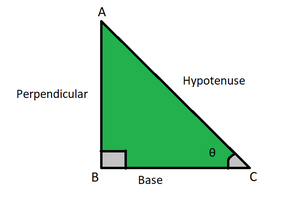If in a Right-angled triangle, AB =Perpendicular, BC = Base and AC = Hypotenuse

Then, (Perpendicular)2 + (Base)2 = (Hypotenuse)2

Therefore, AB2 + BC2 = AC2

This is known as Pythagoras Theorem.

If Sin A = 3/4, Calculate cos A and tan A.

Solution:

Given that, Sin A = 3/4  i.e.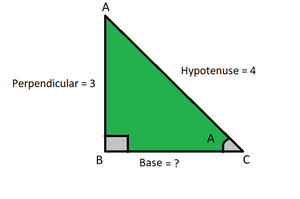For a given problem, let Base = X

Given: AB = Perpendicular = 3 ,  AC = Hypotenuse = 4

Sin(A) = Perpendicular/Hypotenuse = 3/4

Using above Trigonometric ratios:

Cos(A) = Base/Hypotenuse= X/4  ⇢ (equation 1)

Tan(A) = Perpendicular/Base = 3/X  ⇢ (equation 2)

Now to find X we need to apply Pythagoras theorem

Therefore, AB2 + BC2 = AC2

32 + X2 = 42

9 + X2 = 16

X2 = 16 – 9

X 2= 7

X = √ 7.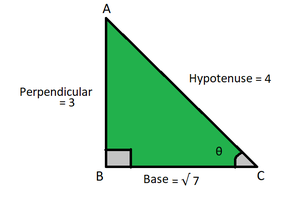Now substituting X in equation 1 and 2 we get:

Cos(A) = Base/Hypotenuse=  √7/4

Tan(A) = Perpendicular/Base = 3/√7

Similar Problems

Question 1: If Sin A = 4/5, Calculate Cot A and Cosec A.

Solution:

Given that, Sin A = 4/5  i.e.

AB = Perpendicular = 4,  AC = Hypotenuse = 5

For a given problem, let Base = X

Sin(A) = Perpendicular/Hypotenuse = 4/5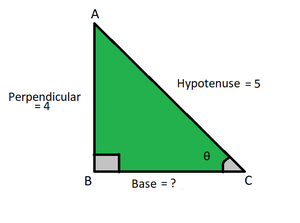Using Trigonometric ratios:

(1) cot (A) = Base/Perpendicular = X/4

Now to find X we need to apply Pythagoras theorem

Therefore, AB2 + BC2 = AC2

42 + BC2 = 52

BC2 = 25 – 16

BC2 = 9

BC = √9

BC = 3

Therefore, cot (A) = Base/Perpendicular = 3/4

(2) cosec (A) = 1/Sin (A) = Hypotenuse/Perpendicular = 5/4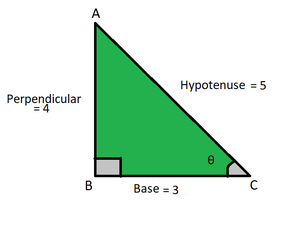Question 2: If Tan = √7/3, Calculate Sec A and Cos A.

Solution:Perpendicular =  √ 7 , Base = 3 , Hypotenuse = ?

Given that Tan A = √7/3

Tan A = Perpendicular/Base

So, Perpendicular = √7, Base = 3

Let Hypotenuse be X

Using Pythagoras theorem

√72 + 32 = X2

72 + 9 = X2

16 = X2

Therefore, X = 4

Hypotenuse = X = 4Perpendicular =  √ 7 , Base = 3 , Hypotenuse = 4

(1) Sec A = Hypotenuse/Base = 4/3

(2) Cos A = 1/Sec A = 3/4

Question 3: If Cot A = √3, Prove that Tan A + Sin A = (2+√3) / 2√3.

Solution:

Given Cot A = √3

Cot A = Base/Perpendicular

So, Base = √3, Perpendicular = 1

Let Hypotenuse be X,Perpendicular =  1 , Base =  √ 3 , Hypotenuse = ?

Using Pythagoras theorem

12 + (√3)2  = X2

1 + 3 = X2

4 = X2

Therefore, X= 2

Hypotenuse = X = 2

Tan A + Sin A = ?

Tan A =1/Cot A = 1/√ 3

Sin A = Perpendicular/Hypotenuse = 1/2

Tan A + Sin A =  1/√3 + 1/2

= (2 + √ 3) / 2√3

Therefore, Tan A + Sin A =  (2 + √3) / 2√3.

Question 4: If cot2 A = 3 (sec2 A – tan2 A) , then find 3(sin A)?

Solution:

Given:  cot2 A = 3 (sec2 A -tan2 A)

sec2 A – tan2 A = 1

Therefore,  cot2 A = 3(1)

So, Cot  A = √3

Cot A =  Base/Perpendicular = √3/1

Base = √3, Perpendicular = 1

Let Hypotenuse be X,Perpendicular =  1 , Base =  √ 3 , Hypotenuse = X

Using Pythagoras theorem

12 + (√3)2 = X2

1 + 3 = X2

4 = X2

Therefore, X = 2

Hypotenuse = X = 2

3(Sin A) = ?

Sin A = Perpendicular/Hypotenuse = 3(Perpendicular/Hypotenuse)

Therefore, Sin A = 3(1/2) = 3/2

Question 5: If sin2A sec A + cos A = 5/4 ,then find tan A?

Solution:

Given: sin2A sec A + cos A = 5/4

Multiply and Divide L.H.S by Cos A ,

Sin2A Sec A + cos A × (Cos A/Cos A) = 5/4

(Sin2 A × Sec A × Cos A + Cos2 A)/Cos A = 5/4

Sec A = 1/Cos A

So, (Sin2 A × 1/ Cos A × Cos A + Cos2 A)/Cos A = 5/4

(Sin2A + Cos2 A)/Cos A = 5/4

1/Cos A = 5/4

Therefore, Cos A = 4/5

Cos A = Base/Hypotenuse = 4/5

Let Perpendicular be XBase = 4 , Hypotenuse = 5 , Perpendicular = X

Using Pythagoras theorem,

42 + X2 = 52

16 + X = 25

X2 = 25 – 16

X2 = 9

X = √9

X = 3

Tan A = ?

Hence, Tan A = Perpendicular/Base = 3/4

My Personal Notes arrow_drop_up